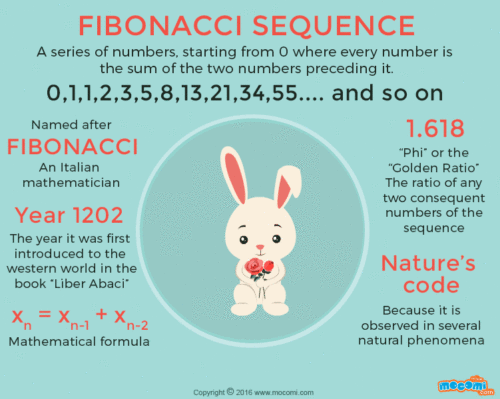# Fibonacci Series In Java Program – 4 Multiple Ways

Java program to display a  Fibonacci Series. We will discuss the various methods to find out the Fibonacci Series In Java Program for the first n numbers. The compiler has been added so that you can execute the set of programs yourself, alongside suitable examples and sample outputs. The methods as aforementioned are:

• Using For Loop.
• Using While Loop.
• Using Static Method.
• Using Recursion.

FIBONACCI SERIES, coined by Leonardo Fibonacci(c.1175 – c.1250) is the collection of numbers in a sequence known as the Fibonacci Series where each number after the first two numbers is the sum of the previous two numbers. The series generally goes like 1, 1, 2, 3, 5, 8, 13, 21 and so on.Image Source

Earlier, 0 was also mentioned making the series 0, 1, 1, 2, 3, 5… The sequence can start with either 1, 1 or 0, 1 irrespective. The Fibonacci Sequence follows the very popular Golden Ratio closely.

## Fibonacci Series In Java – Using For Loop

1) In Fibonacci series each number is addition of its two previous numbers.

2) Read the n value using Scanner object sc.nextInt(), and store it in the variable n.

3) For loop iterates from c=0 to c=n-1.

a) For c=0 nextterm=0, for c=1 nexterm =1

b) For c=2, nextterm=i+j=1(to get next value we are adding previous  two numbers), and “i” initialized to “j” (i.e i=1),”j” initialized to “nextterm” (i.e j=1), for c=3  nextterm =2, for c=4  nextterm=5 .

Output:

### Java Program Display Fibonacci – Using While Loop

1) Read the n value using Scanner object sc.nextInt(), and store it in the variable n.

2) Here  first value=0,second value=1, while loop iterates until i<n is false.

3) For i=0 next=0, for i=1 next=1, for 1=2 next=first+second, and first=second,second=next.

Output:

#### Using Static Method

1) We can call the static method using the class name.

2) Call the series method which is static in the main method.

3) The series method executes the code and prints the series.

Output:

##### Using Recursion

1) In our program fib(int n) method calls itself as fib(–n). Here fib(int n) is the recursive function.

2) After creating an  object to the class “Fibonacci”, call the method using  object as “f.fib(num)”, then fib(int n) will start the execution with n=5 and calls itself with n=n-1, then again method execution started, repeats until the condition “if(n>0)” is false.

Output:

If you have any doubts related to Java program to display Fibonacci series do leave a comment here.

For More Programs Check out Here:

x

## Plus Star Pattern Java Program | Patterns

Java program to print plus star pattern program – We have written the below print/draw ...